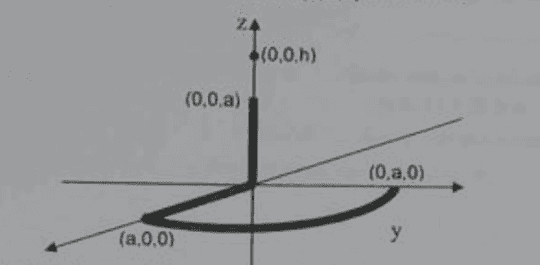# Electric Potential Field Calculation

nownow
Homework Statement:
A finite line of charges has been bent into the shape shown in the figure below (2 orthogonal segments and an arc of a circle). Knowing the charge density in the line is given by ρl (C/m) get the electric potential field at the point (0,0,h). h>a
Relevant Equations:
electric potential field equationI've already tried to calculate the potential with respect to the 3 segments and then apply superposition (V1+V2+V3). However, I was not very successful. My error I think is in the calculation of the radii, mainly of the line segment that is on the z axis. Can anybody help me? I need some light on this question, please

Homework Helper
Gold Member
Homework Statement:: A finite line of charges has been bent into the shape shown in the figure below (2 orthogonal segments and an arc of a circle). Knowing the charge density in the line is given by ρl (C/m) get the electric potential field at the point (0,0,h). h>a
Relevant Equations:: electric potential field equation

View attachment 303063
I've already tried to calculate the potential with respect to the 3 segments and then apply superposition (V1+V2+V3). However, I was not very successful. My error I think is in the calculation of the radii, mainly of the line segment that is on the z axis. Can anybody help me?
We can help you if you show us what you did. Show us your work and where you think you went wrong. Specifically, what radii are you talking about? There is only one radius and that is associated with the quarter-circle. Your superposition approach is correct.

•bob012345
nownow
We can help you if you show us what you did. Show us your work and where you think you went wrong. Specifically, what radii are you talking about? There is only one radius and that is associated with the quarter-circle.
It's not multiple rays. I expressed myself badly, sorry. However, on to my calculations:

$$V_1 = \int_{0}^{a}\frac{\rho_l}{4\pi \varepsilon_0\sqrt{x^2+h^2} }dx$$
$$V_2 = \int_{0}^{a}\frac{\rho_l}{4\pi \varepsilon_0(h-z) }dz$$
$$V_3 = \int_{0}^{\frac{\pi }{2}}\frac{\rho_la}{4\pi \varepsilon_0\sqrt{a^2+h^2} }d\phi$$

Is what I did correct?

Staff Emeritus
Homework Helper
Gold Member
what is the angle between the x and y axis?

nownow
what is the angle between the x and y axis?
The angle is ##\frac{\pi }{2}##

Staff Emeritus
Homework Helper
Gold Member
The angle is ##\frac{\pi }{2}##
So why does your integral specify integration between zero and ##\pi/4##?

Also, editing out an error from your post after getting a reply is not good form.

nownow
I ended up realizing my error shortly after sending the answer. Also, I didn't edit my answer because of your question. Anyway, I apologize for my mistake and I didn't know about this no edit practice, I'm new here. If you want I can edit the question to what it was before, no problem. I just want to clear my doubt on the question. Again sorry for the edit. Thanks

•PeroK and berkeman
Homework Helper
Gold Member
That looks about right. What do you get when you redo the integrals as you have them in post #4 #3? Is your answer the same as you got before?

Last edited:
•Delta2
Homework Helper
Gold Member
post #3 setups correctly the integrals. Now the OP has to calculate them correctly.

•kuruman
Homework Helper
Gold Member
BTW there was another thread, I think from the same OP that the source charge density was portion of a cylindrical surface, so we would have to do a surface integral, I had post to that thread but can't find it anymore, what happened?